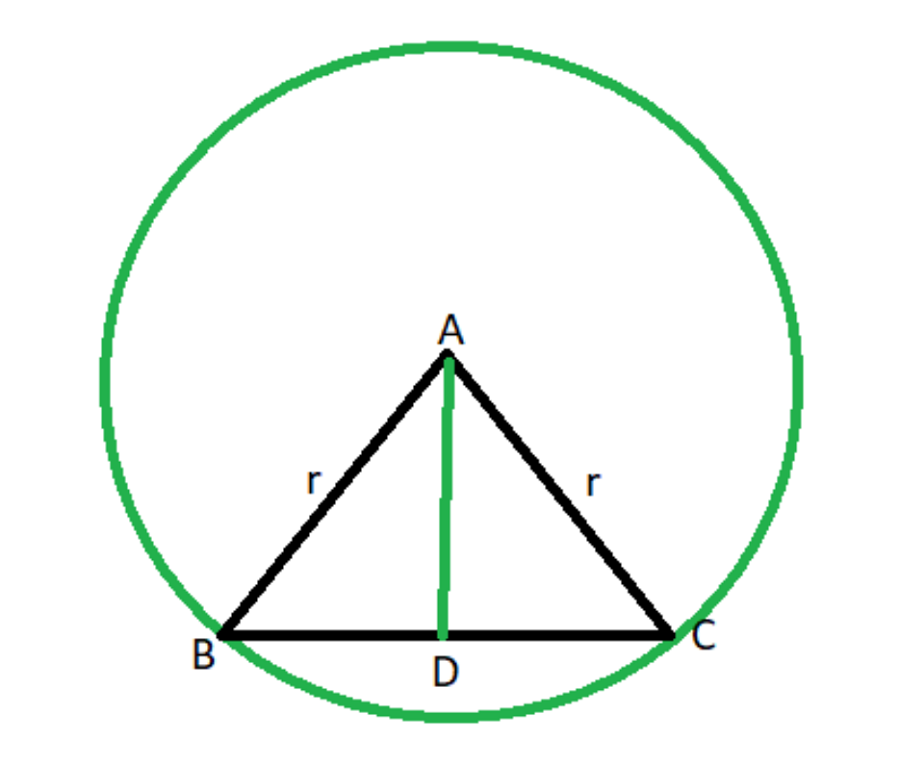GeeksforGeeks App
Open AppBrowser
Continue

# Calculate area and height of an isosceles triangle whose sides are radii of a circle

Given integers R andrepresenting the radius of a circle and the angle formed at the center O by the sector AB (as shown in the figure below), the task is to find the height and area of the triangle formed by connecting the points A, B, and O if possible. Otherwise, print “Not possible”.Examples:

Input: R = 5,= 120
Output:
Height of the triangle is 2.5
Area of triangle is 10.8253
Explanation: The given area and height can be calculated using the equations:
Height =Area =Input: R = 12,= 240
Output: Not possible

Approach: The given problem can be solved based on the following observations:

Observations:• Suppose a perpendicular is drawn on chord AB from point O and the perpendicular cuts the chord at point D. Then the height of the triangle will be OD.>
• According to property of circle the point D divides the chord AB in two equal parts and triangle AOD and BOD will be similar triangle.>
• The angles ∠OAB and ∠OBA are also equal as triangle AOD and BOD are similar and is equal to• The height of the triangle OAB can be calculated using the formula:
•• The area of the triangle can be calculated as:
•Follow the steps below to solve the problem:>

• Check if the angle is greater than 180 or is equal to 0 then print “Not possible”.
• Now convert the angle in radian.
• Calculate the angle ∠OAB and ∠OBA as• Now print the height and area of triangle OAB after calculating it using the above-discussed formula.

Below is the implementation of the above approach:>

## C++

 // C++ program for the above approach #include using namespace std; // Function to convert given// angle from degree to radiandouble Convert(double degree){    double pi = 3.14159265359;    return (degree * (pi / 180));} // Function to calculate height// and area of the triangle OABvoid areaAndHeightOfTraingle(    double radius,    double a){    if (a >= 180 || a == 0) {        cout << "Not possible";        return;    }     // Stores the angle OAB and OBA    double base_angle = (180 - a) / 2;     // Stores the angle in radians    double radians = Convert(base_angle);     // Stores the height    double height = sin(radians) * radius;     // Print height of the triangle    cout << "Height of triangle "         << height << endl;     // Stores the base of triangle OAB    double base = cos(radians) * radius;     // Stores the area of the triangle    double area = base * height;     // Print the area of triangle OAB    cout << "Area of triangle "         << area << endl;} // Driver Codeint main(){    double R = 5, angle = 120;    areaAndHeightOfTraingle(R, angle);     return 0;}

## Java

 // Java program for the above approachimport java.util.*;class GFG{   // Function to convert given  // angle from degree to radian  static double Convert(double degree)  {    double pi = 3.14159265359;    return (degree * (pi / 180));  }   // Function to calculate height  // and area of the triangle OAB  static void areaAndHeightOfTraingle(    double radius, double a)  {    if (a >= 180 || a == 0)    {      System.out.println("Not possible");      return;    }     // Stores the angle OAB and OBA    double base_angle = (180 - a) / 2;     // Stores the angle in radians    double radians = Convert(base_angle);     // Stores the height    double height = Math.sin(radians) * radius;     // Print height of the triangle    System.out.println("Height of triangle " + height);     // Stores the base of triangle OAB    double Base = Math.cos(radians) * radius;     // Stores the area of the triangle    double area = Base * height;     // Print the area of triangle OAB    System.out.println("Area of triangle " + area);  }    // Driver Code  public static void main(String[] args)  {    double R = 5, angle = 120;    areaAndHeightOfTraingle(R, angle);  }} // This code is contributed by sanjoy_62.

## Python3

 # Python3 program for the above approachfrom math import sin,cos # Function to convert given# angle from degree to radiandef Convert(degree):    pi = 3.14159265359    return (degree * (pi / 180)) # Function to calculate height# and area of the triangle OABdef areaAndHeightOfTraingle(radius, a):    if (a >= 180 or a == 0):        print("Not possible")        return     # Stores the angle OAB and OBA    base_angle = (180 - a) / 2     # Stores the angle in radians    radians = Convert(base_angle)     # Stores the height    height = sin(radians) * radius     # Print height of the triangle    print("Height of triangle ", round(height, 1))     # Stores the base of triangle OAB    base = cos(radians) * radius     # Stores the area of the triangle    area = base * height     # Print the area of triangle OAB    print("Area of triangle ", round(area, 4)) # Driver Codeif __name__ == '__main__':    R , angle = 5, 120    areaAndHeightOfTraingle(R, angle)         # This code is contributed by mohit kumar 29.

## C#

 // C# program for the above approachusing System;public class GFG{     // Function to convert given    // angle from degree to radian    static double Convert(double degree)    {        double pi = 3.14159265359;        return (degree * (pi / 180));    }         // Function to calculate height    // and area of the triangle OAB    static void areaAndHeightOfTraingle(        double radius, double a)    {        if (a >= 180 || a == 0)        {            Console.WriteLine("Not possible");            return;        }             // Stores the angle OAB and OBA        double base_angle = (180 - a) / 2;             // Stores the angle in radians        double radians = Convert(base_angle);             // Stores the height        double height = Math.Sin(radians) * radius;             // Print height of the triangle        Console.WriteLine("Height of triangle " + height);             // Stores the base of triangle OAB        double Base = Math.Cos(radians) * radius;             // Stores the area of the triangle        double area = Base * height;             // Print the area of triangle OAB        Console.WriteLine("Area of triangle " + area);    }     // Driver Code    static public void Main ()    {        double R = 5, angle = 120;        areaAndHeightOfTraingle(R, angle);    }} // This code is contributed by AnkThon

## Javascript

 

Output:

Height of triangle 2.5
Area of triangle 10.8253

Time Complexity: O(1)
Auxiliary Space: O(1)

My Personal Notes arrow_drop_up